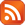CATEGORIES# How to Code and Decode an URL

FEB 5 2008

I am passing my variable values in URL so I need to encode and decode it properly. I don't want to pass some character where page not found pops up as a 404 error.

## COMMENT

Name: Nisa

You can use vbscript built-in function for CODE and customize function for DECODE.
ENCODE: Server.URLEncode(display)
DECODE: Function URLDecode(sDecode)
Dim aSplit
Dim oDecode
Dim I
If IsNull(sDecode) Then
URLDecode = ""
Exit Function
End If
' replace all pluses to spaces
oDecode = REPLACE(sDecode, "+", " ")
' convert %hexdigits to character
aSplit = Split(oDecode, "%")
If IsArray(aSplit) Then
oDecode = aSplit(0)
For I = 0 to UBound(aSplit) - 1
oDecode = oDecode & _
Chr("&H" & Left(aSplit(i + 1), 2)) &_
Right(aSplit(i + 1), Len(aSplit(i + 1)) - 2)
Next
End If
URLDecode = oDecode
End Function

 *Name: *Comment:You can use HTML tags here. *Code: Please enter the sum of 5+2